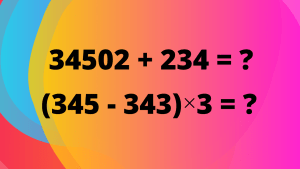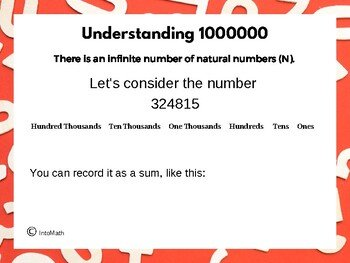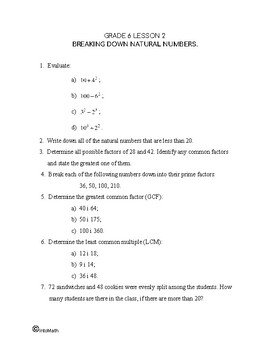Video LessonCA$2.00 We have already learnt about Natural numbers in our grade 5 lessons. Now we are moving on to working with much larger numbers. Sometimes we need to use really large numbers when measuring distances or calculating weight of a big object. What if we wanted to calculate the distance around the Earth? One million (1000,000) is a very large number. In this lesson you will learn and practice how to add and subtract numbers up to 1000,000, as well as how to multiply and divide them using a variety of strategies and methods (breaking the number down by place value of its digits, vertical multiplication, long division). For example, you will see how breaking the large numbers down by place value of the digits in each number helps to quickly add and subtract the two numbers. In 234,560 + 345,678, add 200,000 and 300,000, then 30,000 + 40,000 and so on..Grade 6 Workbook (16 pages with answers) CA$4.00

When multiplying and dividing large numbers, we often use vertical multiplication and long division. Thus, it is important to be comfortable with conducting these operations with large numbers.

This lesson also discusses the order of operations, BEDMAS (Brackets, Exponents, Division, Multiplication, Addition, Subtraction), which is a very useful concept in every mathematics course.

It is always important to remember that inverse operations, such as addition and subtraction or multiplication and division are done whichever comes first in the mathematical expression from left to right.

For example, in the expression 230 × (3 +123,456) ÷ 3 even though there are both division and multiplication and in BEDMAS acronym division comes first, in this case multiplication is the first operation if you read the expression from left to right. Therefore, after performing the operation in brackets, multiply the result by 230 and then divide the product by 3 to get the final answer.